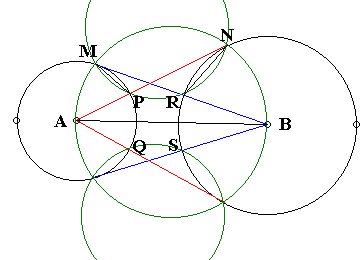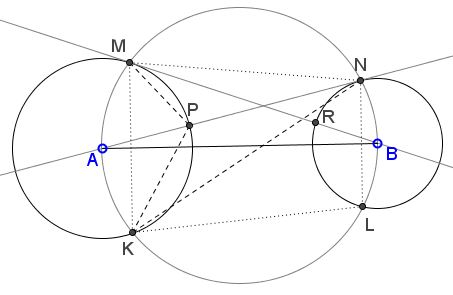# The Eyeball Theorem

### The Eyeball Theorem

Let there be two circles $C(A, R_{A})$ and $C(B, R_{B}),$ one with center $A$ and radius $R_{A},$ the other with center $B$ and radius $R_{B}.$ Assume the tangents from $A$ to $C(B, R_{B})$ intersect $C(A, R_{A})$ at points $P$ and $Q,$ whereas the tangents from $B$ to $C(A, R_{A})$ intersect $C(B, R_{B})$ at points $R$ and $S.$Then $PQ = RS.$

### Proof 1Angles $AMB$ and $ANB$ are right. Therefore, the quadrilateral $AMNB$ is cyclic, which implies

(1)

$\angle MAN = \angle MBN,$

since the two angles subtend the same arc. Now,

$\angle PMR = \angle MAP/2 = \angle MAN/2\\ \angle PNR = \angle RBN/2 = \angle MBN/2$

since the two angles are formed by a tangent and a chord. Along with (1) this gives

(2)

$\angle PMR = \angle PNR,$

which means that the quadrilateral $MPRN$ is also cyclic. From here,

(3)

$\angle NMR = \angle NPR,$

while, for a similar reason in the circle circumscribing the quadrilateral $AMNB,$

(4)

$\angle NAB = \angle NMB = \angle NMR,$

so that from (3) and (4) we conclude that

$\angle NPR = \angle NAB.$

In other words, $PR$ is parallel to $AB.$ Since $PQ \perp AB$ and $RS \perp AB$, and because of the symmetry in $AB,$ the quadrilateral $PQSR$ is a rectangle, so that $PQ = RS,$ indeed.

### Proof 2

(NRich Program, University of Cambridge)Let $C$ be the midpoint of $PQ.$ $C$ lies on $AB,$ and therefore $\Delta ACP$ is right. As such, it is similar to the right triangle $ANB,$ since the two share an angle. From the similarity of the triangles we derive the proportion $CP/AP = BN/AB,$ which means that

(5)

$CP = R_{A}R_{B}/AB,$ or $PQ = 2\cdot R_{A}R_{B}/AB.$

The latter expression is symmetric in $A$ and $B.$ Therefore also $RS = 2\cdot R_{A}R_{B}/AB.$ Q.E.D.

### Proof 2'

We could have used (5) a little differently. Assume the circle $C(A, R_{A})$ is fixed as is the point $B.$ What happens when $R_{B}$ changes? Obviously (or, e.g., because all circles are similar), $DR,$ where $D$ is the center of $RS,$ changes linearly in proportion to $R_{B}.$ From (5), the same is true of $CP.$ The rates are bound to be the same, since when $R_{A} = R_{B},$ we trivially have $CP = DR.$ Therefore, the latter identity holds for any $R_{B}.$

### Proof 3

(E. J. Barbeau, M. S. Klamkin, W. O. J. Moser, Problem 185)Triangles $AMU$ and $BNU$ are similar, from which

(6)

$AM/AU = BN/BU$

On the other hand, $AM = AP$ and $BN = BR,$ such that (6) implies

(6')

$AP/AU = BR/BU$

Because of the symmetry of the configuration, $UV$ is orthogonal to $AB$ and is, therefore, parallel to $PQ$ and $RS.$ From here triangles $AUV$ and $APQ$ are similar as are triangles $BUV$ and $BRS.$ In combination with (6'), we obtain

$PQ/UV = AP/AU = BR/BU = RS/UV,$

which simply means that $PQ = RS.$

### Proof 3'

Floor van Lamoen has suggested a different ending following (6'). The latter says that $PR$ is parallel to $AB$ as $P$ and $R$ divide the sides $AU$ and $BU$ of triangle $ABU$ in the same ratio. The same holds for $QS:$ $QS\parallel AB.$ It follows that $QSRP$ is a rectangle which proves the theorem.

Floor also came up with an entirely novel proof, which, although, lengthy brings to light additional features of the configuration, see Proof 4.

### Proof 4

(By Floor van Lamoen, March 2006, private communication)Extend $PR$ to $TU$ with $T$ on circle $A$ and $U$ on circle $B.$ Let $TM$ and $UN$ meet in $V.$

Note that $\angle MAN = \angle MBN$ and thus

$\angle VTU = \angle MTP = \frac{1}{2}\angle MAN = \frac{1}{2}\angle MBN = \angle RUN = \angle VUT,$

so triangle $TUV$ is isosceles with $VT = VU.$

We also see that

$\angle MBN = \angle VTU + \angle TUV$

so that $\angle MBN$ and $\angle NVM$ add to $180^{\circ}$, implying that $V$ lies on the circle with diameter $AB.$

Since inscribed angles $TVA$ and $KVA$ subtend equal arcs they are equal: $\angle TVA = \angle KVA$ and, similarly, $\angle UVB = \angle LVB$. By reflection through $AV$ and $BV$ respectively, we see that in fact $VT = VK = VL = VU.$

Let $W$ be the reflection of $V$ through $AB.$ $\Delta WMN$ is isosceles just as $\Delta KLV.$ Hence

$\angle WVN = \angle WMN = \angle WNM = \angle WVM,$

so $VW$ bisects angle $TVU,$ and $VW$ is perpendicular to $PR,$ and thus $PR$ is parallel to $AB.$ By symmetry $PQSR$ is a rectangle.

### Proof 5

Talk about the length of a proof. Here is quite a short one also by Floor van Lamoen (private communication, March 28, 2006):

With a reference to the following diagramNote that $\angle RNA = \angle RTN$ by inscribed angles.

By isosceles $\Delta NBR,$ $\angle BNR = \angle BRN.$

So

\begin{align} \angle RTN + \angle TRN &= \angle RTN + \angle BRN\\ &= \angle RNA + \angle BNR\\ &= 90^{\circ}. \end{align}

This shows that $TR$ is a diameter of circle $(B).$ Hence $\angle RST$ is right. By symmetry $PQRS$ is a rectangle.

Remark: This proof makes an unwarranted assumption. Can you locate it?

### Proof 6

Dao Thanh Oai has noticed that the Eyeball theorem is a consequence of a Japanese sangaku, concerning a convex quadrilateral $ABCD.$ If the quadrilateral is cyclic, the incenters of triangles $BCD,$ $ACD,$ $ABD,$ and $ABC$ form a rectangle. As applied to the Eyeball theorem, the quadrilateral in question is $MKLN$ whereas points $P,$ $Q,$ $R,$ and $S$ are the incenters of the corresponding triangles. I'll show that this is true of $P$ and $\Delta MKN.$ Three other points are treated similarly.Indeed, in circle $(AB),$ $\angle MAN=\angle MKN.$ In circle $(A),$ $\angle MKP=\frac{1}{2}\angle MAP=\frac{1}{2}\angle MAN.$ It follows that $\angle MKP=\angle PKN$ as both equal half of $\angle MKN.$ This means that $KP$ is the angle bisector of $\angle MKN.$

Points $M$ and $K$ are symmetric in $AB,$ implying that arcs $MA$ and $AK$ of circle $(AB)$ are equal, so that too $\angle MNA=\angle ANK,$ which shows that NP is the bisector of $\angle MNK,$ making $P$ the incenter of $\Delta MKN.$

### Remark

There is another interesting fact with ophthalmological connotations that I dubbed the Squinting Eyes Theorem. There are actually several more, see the list at the bottom of the page.

### References

1. E. J. Barbeau, M. S. Klamkin, W. O. J. Moser, Five Hundred Mathematical Challenges, MAA, 1995
2. J. Konhauser, D. Velleman, S. Wagon, Which Way Did the Bicycle Go?, MAA, 1996, #33
3. D. Wells, Curious and Interesting Geometry, Penguin Books, 1991### Problems with Ophthalmological Connotations

• Eye-to-Eye Theorem I
• Eye-to-Eye Theorem II
• The Squinting Eyes Theorem
• Eyeballing a ball
• Praying Eyes Theorem
• Focus on the Eyeball Theorem
• Eyeball Theorem Rectified
• Bespectacled Eyeballs Extension
• Shedding Light on the Ball for Eyeballing
• Eyeballs Projected
• Archimedean Siblings out of Wedlock, i.e., Arbelos
• Rectified, Halved, Sheared, Eyeballs Still Surprise
•